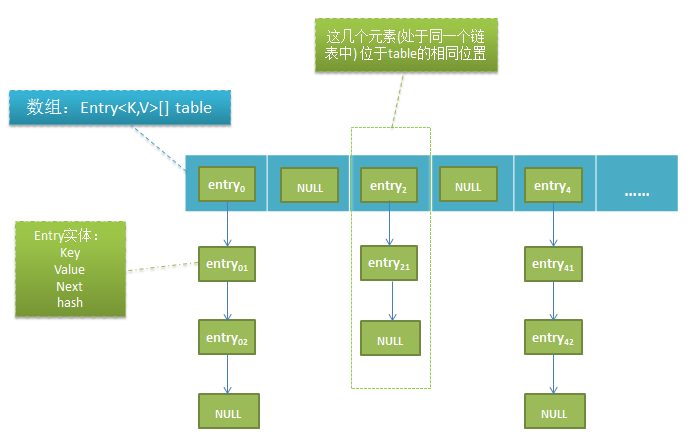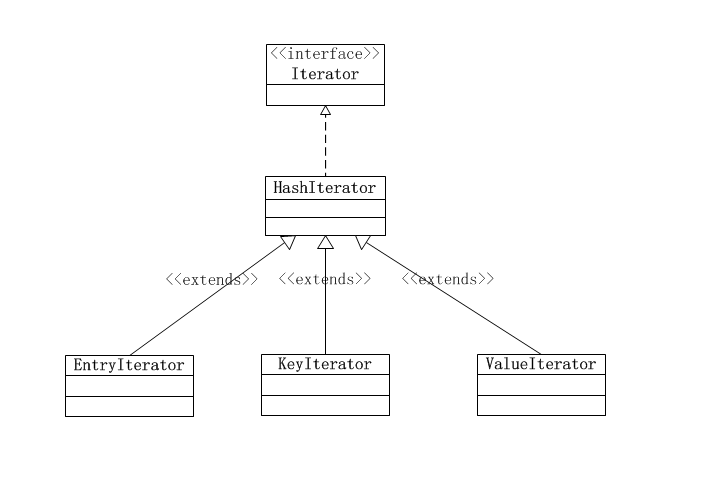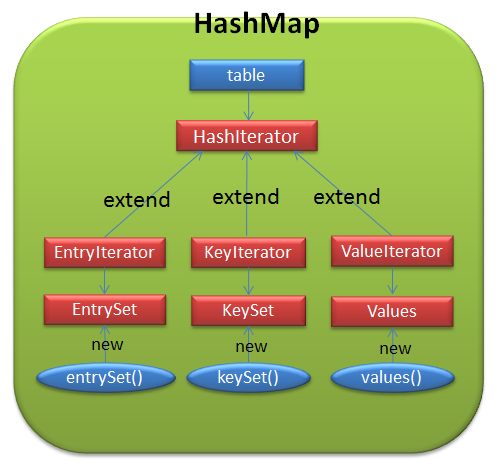# java集合-HashMap

## 1. HashMap特点

HashMap基于哈稀表(或者叫哈希表，不是指Hashtable)实现Map接口，它允许为null的key以及value，而且不保证映射的顺序，毕竟当容量到阈值时会进行扩容，原map中的所有元素会被重哈稀。HashMap经常拿来跟Hashtable进行对比，前者除了不同步以及允许null值外，其它大致上都跟后者差不多

HashMap class is roughly equivalent to Hashtable, except that it is unsynchronized and permits nulls

## 2. 主要属性

static final int DEFAULT_INITIAL_CAPACITY = 1 << 4; // 默认初始容量：16，用位运算提高效率

static final int MAXIMUM_CAPACITY = 1 << 30;//最大容量，2^30=1073741824

static final float DEFAULT_LOAD_FACTOR = 0.75f; //默认加载因子
transient Entry<K,V>[] table = (Entry<K,V>[]) EMPTY_TABLE;  //大小总是为2^n

transient int size;  //hashmap中当前元素数量

transient int modCount; //hashmap在结构上被修改的次数，在迭代时，如果expectedModCount!=modCount则会快速失败

## 3. 数据结构## 4. 方法hash、indexFor

static int indexFor(int h, int length) {
//h为hashCode,length必须为2的n次方
return h & (length-1);  //返回按位与的结果
}

## 5. 添加元素

 public V put(K key, V value) {
if (table == EMPTY_TABLE) {  //table还没初始化
inflateTable(threshold); //给table分配空间
}
if (key == null)  //对于key==null的元素统一调用puForNullKey
return putForNullKey(value);
int hash = hash(key);  //根据key计算元素的hashCode
int i = indexFor(hash, table.length);  //根据hashCode计算bucketIndex
for (Entry<K,V> e = table[i]; e != null; e = e.next) {
Object k;
//hashCode相等是key相同的前提
if (e.hash == hash && ((k = e.key) == key || key.equals(k))) {
V oldValue = e.value;
e.value = value;
e.recordAccess(this);
return oldValue;
}
}  //从链表头table[i]开始遍历链表，并对比第一个元素，如果存在相同key的元素，则更新元素，返回旧元素，退出put方法
modCount++;  //将会往hashmap中添加一个新的元素，属于结构上的修改
return null;
}

1.先判断table == EMPTY_TABLE，如果相等的话，则先初始化table，分配空间

private void inflateTable(int toSize) {
// roundUpToPowerOf2方法返回一个:
//1.比toSize大
//2.是2的次方
//3.最接近toSize
int capacity = roundUpToPowerOf2(toSize);

threshold = (int) Math.min(capacity * loadFactor, MAXIMUM_CAPACITY + 1);
table = new Entry[capacity];
initHashSeedAsNeeded(capacity);  //重新计算hashSeed的值
}

2.我们知道，HashMap是允许key和value为null的，对于key==null的元素调用putForNullKey

private V putForNullKey(V value) {
for (Entry<K,V> e = table; e != null; e = e.next) {
if (e.key == null) {
V oldValue = e.value;
e.value = value;
e.recordAccess(this);
return oldValue;
}
}  //从table处的链表遍历，如果找到key==null的元素，则更新值
modCount++;
return null;
}

3.通过hash方法计算出hashCode后，利用indexFor方法找到对应的table中的位置i

4.从链表头table[i]开始遍历，判断是否存在相同的key元素，如果重新，则更新value，返回旧值并终止操作，否则，进入步骤5。从源码中可以看出，判断key是否相同不仅要比较两key的值，而且还要对hashCode进行对比。

5.调用addEntry方法来添加指定key,value的元素，它负责判断并进行重新调整数组table的大小

void addEntry(int hash, K key, V value, int bucketIndex) {
//如果hashmap的元素数量(size)已经到到阀值
//或者将要添加的元素的桶位置不为空，表示需要额外的空间
if ((size >= threshold) && (null != table[bucketIndex])) {
resize(2 * table.length); //调整table大小为原本的2倍
hash = (null != key) ? hash(key) : 0;  //计算hashCode
bucketIndex = indexFor(hash, table.length);  //计算桶索引
}

createEntry(hash, key, value, bucketIndex);  //创建一个新的元素实体并保存到相应位置
}

6.调用createEntry方法构造元素(Entry实体)，并保存

    void createEntry(int hash, K key, V value, int bucketIndex) {
Entry<K,V> e = table[bucketIndex];  //先记录新元素即将要保存的位置的元素
table[bucketIndex] = new Entry<>(hash, key, value, e); //构造出新元素，保存到table[bucketIndex]，新元素的next为e
size++;
}

## 6. 扩容、重哈稀

void resize(int newCapacity) {  //newCapacity必需是2^n，除非已经到达了MAXIMUM_CAPACITY
Entry[] oldTable = table;
int oldCapacity = oldTable.length;
if (oldCapacity == MAXIMUM_CAPACITY) {
threshold = Integer.MAX_VALUE;
return;
}//如果容量已经到达了最大，那么不能再扩容

Entry[] newTable = new Entry[newCapacity]; //根据新容量创建新的数组
transfer(newTable, initHashSeedAsNeeded(newCapacity)); //把旧数组的元素转换到新的数组
table = newTable;
threshold = (int)Math.min(newCapacity * loadFactor, MAXIMUM_CAPACITY + 1);
}

javadoc中强调了resize方法的参数必须为2的n次方，而且要比当前的容量大，除非容量已经达到了最大值MAXIMUM_CAPACITY

newCapacity the new capacity, MUST be a power of two; must be greater than current capacity unless current capacity is MAXIMUM_CAPACITY

• 当length=16时
hashCode操作length-1=结果(bucketIndex)
1&15=1
3&15=2
3&15=3
4&15=4
5&15=5
6&15=6
7&15=7
8&15=8
9&15=9
10&15=10
11&15=11
12&15=12
13&15=13
14&15=14
15&15=15

* 当length=15时

hashCode操作length-1=结果(bucketIndex)
1&15=0
3&15=2
3&15=2
4&15=4
5&15=4
6&15=6
7&15=6
8&15=8
9&15=8
10&15=10
11&15=10
12&15=12
13&15=12
14&15=14
15&15=14

// Find a power of 2 >= toSize
int capacity = roundUpToPowerOf2(toSize);  //如果toSize=15,则会得到capacity=16，具体的实现有兴趣可以看源码

void transfer(Entry[] newTable, boolean rehash) {
int newCapacity = newTable.length;
for (Entry<K,V> e : table) {  //遍历数组元素
while(null != e) {  //每个数组元素都是一个链表的表头，从表头开始遍历该链表
Entry<K,V> next = e.next;
if (rehash) {
e.hash = null == e.key ? 0 : hash(e.key);  //重新计算hashCode
}
int i = indexFor(e.hash, newCapacity);  //重新计算桶索引bucketIndex
e.next = newTable[i];
newTable[i] = e; //将元素e插入newtable[i]
e = next;
}
}
}

## 7. 迭代器

HashMap内部有3种迭代器:EntryIterator,KeyIterator,ValueIterator，它们的继承关系如下图：1. EntryIterator：可以迭代map中所有元素实体
2. KeyIterator：可以迭代map中所有元素实体的key值
3. ValueIterator：可以迭代map中所有元素实体的value值

EntryIterator –>EntrySet extends AbstractSet<Map.Entry<K,V>>

KeyIterator–>KeySet extends AbstractSet<Map.Entry<K,V>>

ValueIterator–>Values extends AbstractCollection

EntrySet : a Set view of the mappings contained in this map
KetSet : a Set view of the keys contained in this map
Values : a Collection view of the values contained in this map## 9. 参考

10-14294
03-211万+
08-24254
07-20346
02-011205
01-31469
03-10663
03-131240
03-131164
09-28264
11-22250
11-071万+
01-057261
03-101131
09-09692
08-30

### “相关推荐”对你有帮助么？

•非常没帮助
•没帮助
•一般
•有帮助
•非常有帮助被折叠的  条评论 为什么被折叠?到【灌水乐园】发言¥2 ¥4 ¥6 ¥10 ¥20余额支付 (余额：-- )扫码支付获取中扫码支付点击重新获取扫码支付1.余额是钱包充值的虚拟货币，按照1:1的比例进行支付金额的抵扣。
2.余额无法直接购买下载，可以购买VIP、C币套餐、付费专栏及课程。余额充值